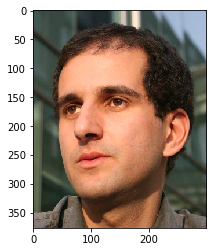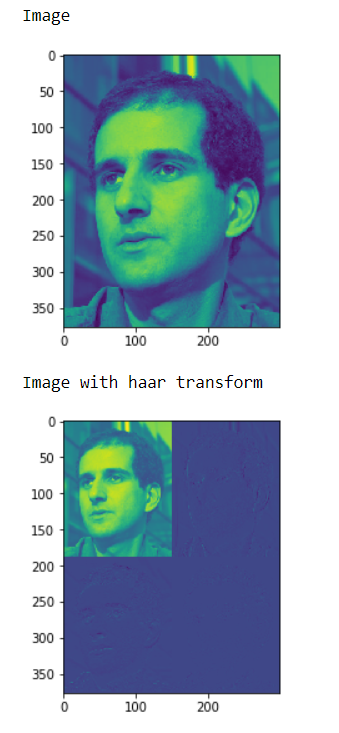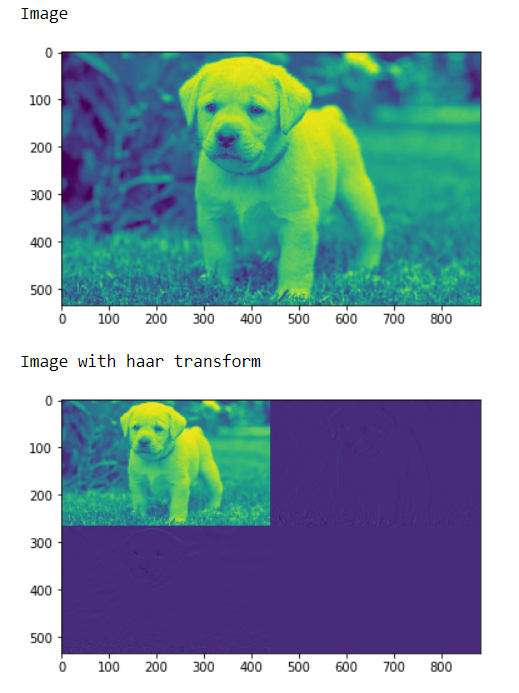# Mahotas – Haar Transform

• Last Updated : 10 Jun, 2021

In this article we will see how we can do image haar transform in mahotas. The haar wavelet is a sequence of rescaled “square-shaped” functions which together form a wavelet family or basis. Wavelet analysis is similar to Fourier analysis in that it allows a target function over an interval to be represented in terms of an orthonormal basis. The Haar sequence is now recognised as the first known wavelet basis and extensively used as a teaching example.
In this tutorial we will use “luispedro” image, below is the command to load it.

`mahotas.demos.load('luispedro')`

Below is the luispedro imageIn order to do this we will use mahotas.haar method

Syntax : mahotas.haar(img)
Argument : It takes image object as argument
Return : It returns image object

Note : Input image should be filtered or should be loaded as grey
In order to filter the image we will take the image object which is numpy.ndarray and filter it with the help of indexing, below is the command to do this

`image = image[:, :, 0]`

Example 1:

## Python3

 `# importing various libraries``import` `numpy as np``import` `mahotas``import` `mahotas.demos``from` `mahotas.thresholding ``import` `soft_threshold``from` `pylab ``import` `imshow, show``from` `os ``import` `path` `# loading image``f ``=` `mahotas.demos.load(``'luispedro'``, as_grey ``=` `True``)`  `# showing image``print``(``"Image"``)` `imshow(f)``show()` `# haar transform``h ``=` `mahotas.haar(f)` `# showing image``print``(``"Image with haar transform"``)``imshow(h)``show()`

Output :Example 2:

## Python3

 `# importing required libraries``import` `mahotas``import` `numpy as np``from` `pylab ``import` `imshow, show``import` `os`  `# loading image``img ``=` `mahotas.imread(``'dog_image.png'``)` `# filtering image``img ``=` `img[:, :, ``0``]`  `# showing image``print``(``"Image"``)` `imshow(img)``show()` `# haar transform``h ``=` `mahotas.haar(img)` `# showing image``print``(``"Image with haar transform"``)``imshow(h)``show()`

Output :My Personal Notes arrow_drop_up